Function Repository Resource:

# GraphPathMatrix

Find the path matrix of a graph

Contributed by: Peter Burbery
 ResourceFunction["GraphPathMatrix"][g] gives the path matrix of the graph g.

## Details

An entry pij of the path matrix is 1 if there is a path between vertex νi and vertex νj, and 0 otherwise.
The path matrix is related to the Floyd-Warshall algorithm.
ResourceFunction["GraphPathMatrix"][g,SparseArray] gives the path matrix as a SparseArray object.
An undirected connected graph will have all matrix entries equal to 1. These are not terribly interesting. The function is better suited to directed graphs where not all components are connected rather than to graphs where every vertex is reachable.

## Examples

### Basic Examples (2)

Find the path matrix representation of a directed graph:

 In:=Out=A random graph:

 In:=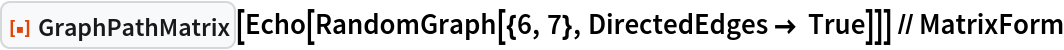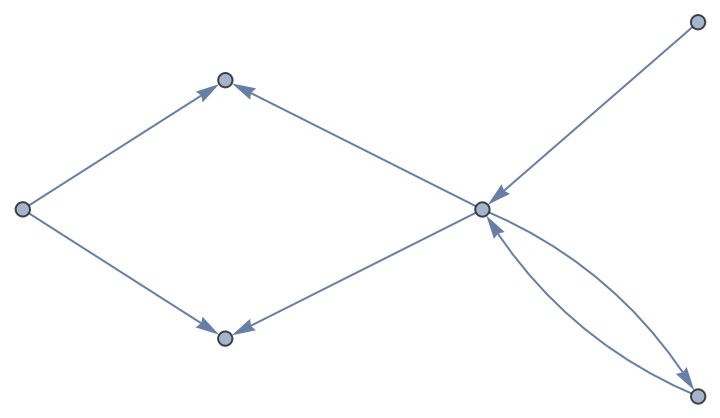Out=### Scope (13)

A directed graph generated from the Price graph distribution:

 In:=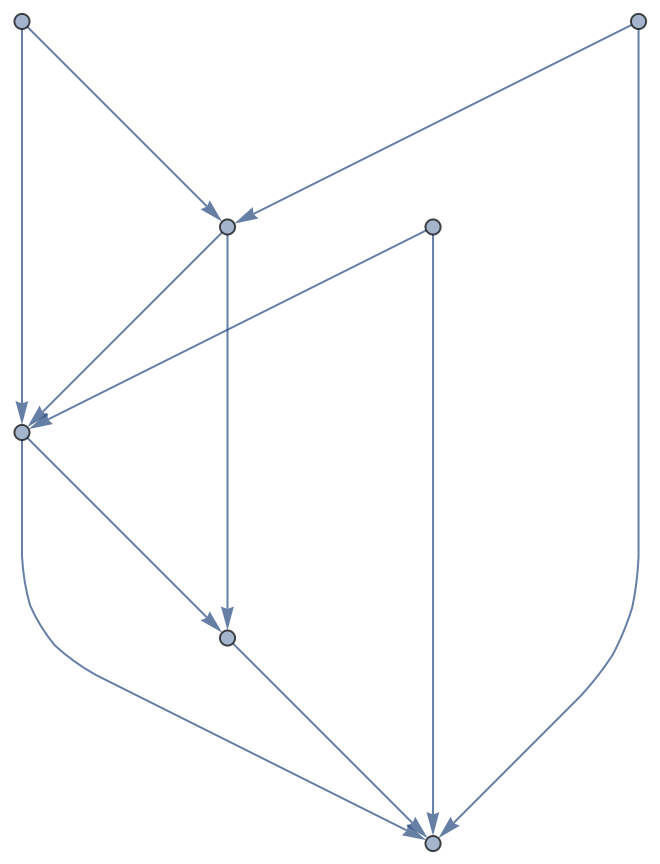Out=Random cycle graph:

 In:=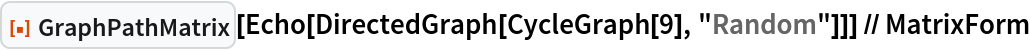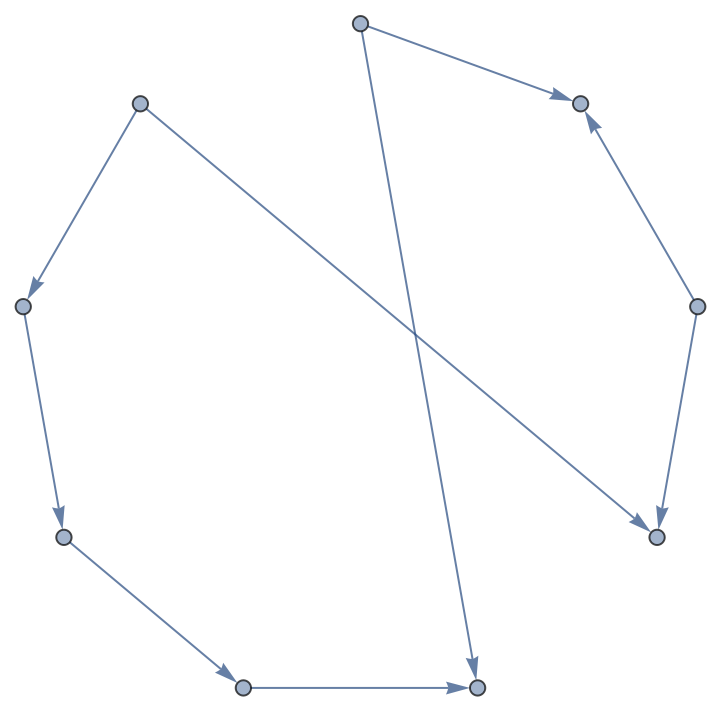Out=Directed graph from graph product:

 In:=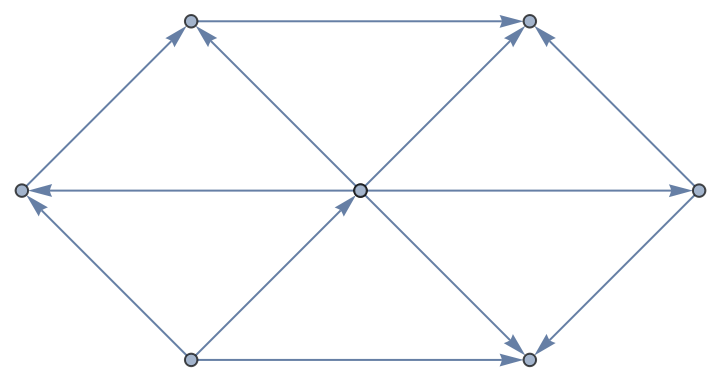Out=Directed graph from graph product of multigraphs:

 In:=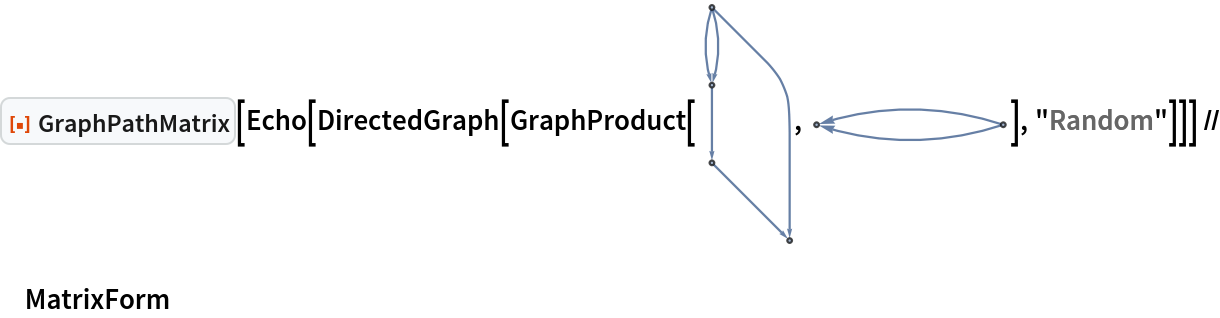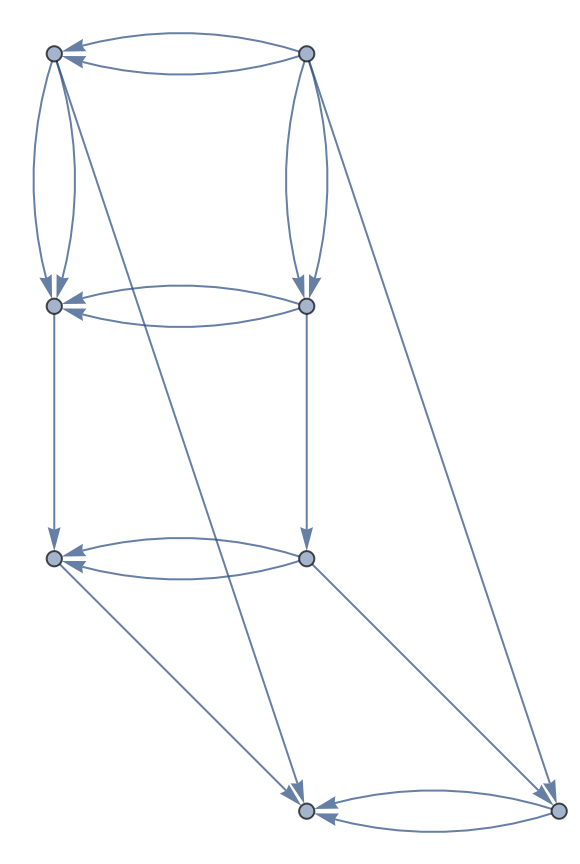Out=A parametric k-ary tree graph:

 In:=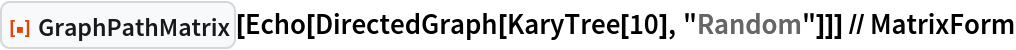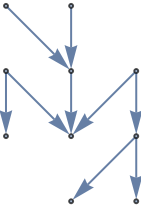Out=A parametric complete k-ary tree graph:

 In:=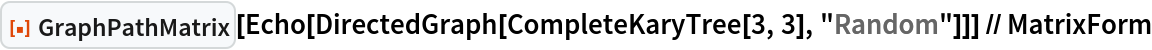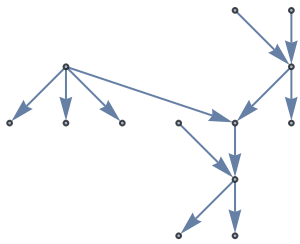Out=A random directed graph:

 In:=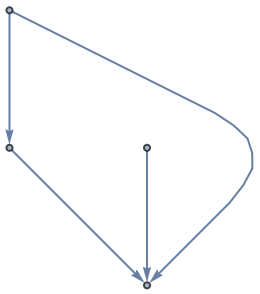Out=An acyclic directed graph:

 In:=Out=Another acyclic directed graph:

 In:=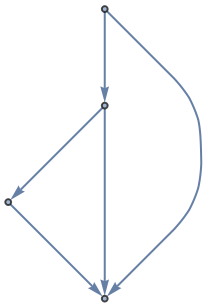Out=A random Bernoulli graph:

 In:=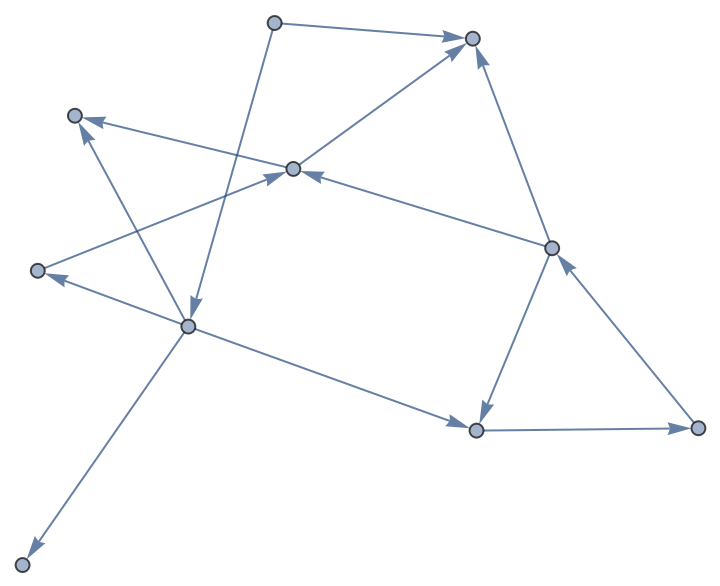Out=A random disconnected spatial directed graph with random orientations for the directed edges:

 In:=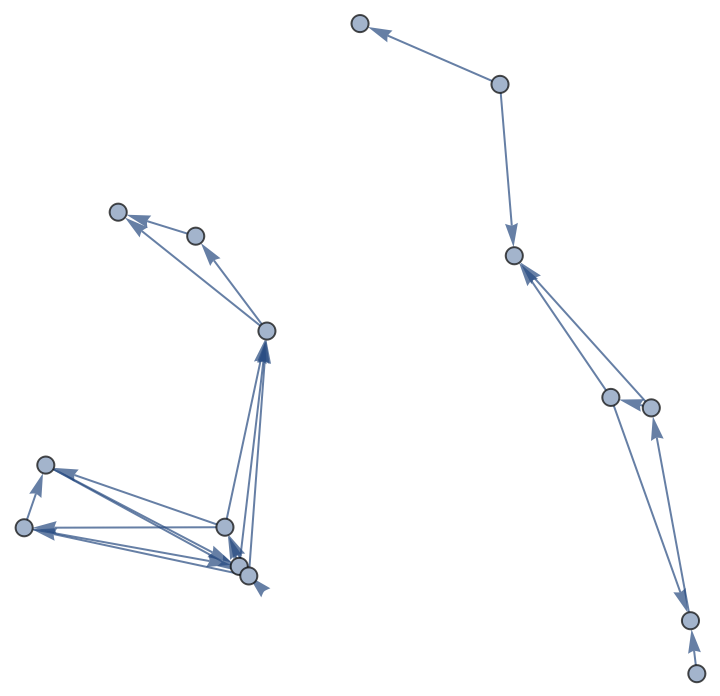Out=Find the path matrix representation of the Petersen graph with script capital letters for the vertex names:

 In:=Out=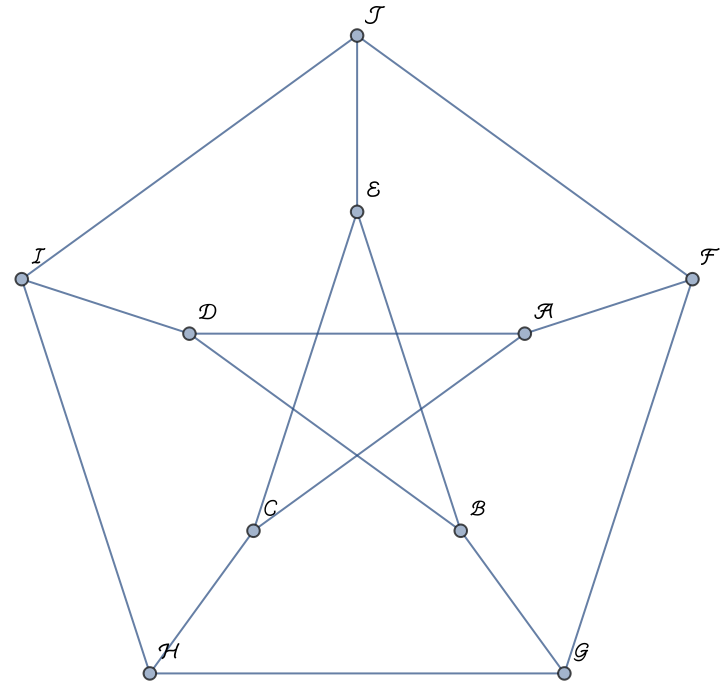Find the path matrix representation of the undirected graph:

 In:=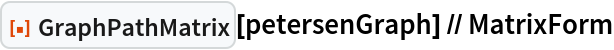Out=Output the matrix as a sparse array for efficiency:

 In:=Out=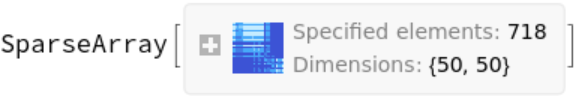Peter Burbery

## Requirements

Wolfram Language 13.2 (December 2022) or above

## Version History

• 1.0.0 – 02 June 2023

## Author Notes

The function often generates a matrix with all 1s.